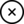## Electromagnetic Induction

Discovered in 1831 by Michael Faraday, and independantly again by Joseph Henry in 1832. Electromagnetic Induction is the Time Rate of Change (Delta t) of the Magnetic Field defined as (Delta ϕ (Phi) ), in the Proximity of a Conductor.

Thus the Equation is: E.M.F = -N dϕ/dt

Where:

• E.M.F = The Electromotive Force, the difference in Potential Charge, measured in Volts.
• - (Negative Sign) = Lenz's Law.
• N = The Number of Turns.
• dϕ = Rate of Change of the Magnetic Field.
• dt = Rate of Change of Time.